The NLIN Procedure

Example 69.2 Iteratively Reweighted Least Squares

With the NLIN procedure you can perform weighted nonlinear least squares regression in situations where the weights are functions of the parameters. To minimize a weighted sum of squares, you assign an expression to the _WEIGHT_ variable in your PROC NLIN statements. When the _WEIGHT_ variable depends on the model parameters, the estimation technique is known as iteratively reweighted least squares (IRLS). In this situation you should employ the NOHALVE option in the PROC NLIN statement. Because the weights change from iteration to iteration, it is not reasonable to expect the weighted residual sum of squares to decrease between iterations. The NOHALVE option removes that restriction.

Examples where IRLS estimation is used include robust regression via M-estimation (Huber, 1964, 1973), generalized linear models (McCullagh and Nelder, 1989), and semivariogram fitting in spatial statistics (Schabenberger and Pierce, 2002, Sect. 9.2). There are dedicated SAS/STAT procedures for robust regression (the ROBUSTREG procedure) and generalized linear models (the GENMOD and GLIMMIX procedures). Examples of weighted least squares fitting of a semivariogram function can be found in Chapter 109: The VARIOGRAM Procedure.

In this example we show an application of PROC NLIN for M-estimation only to illustrate the connection between robust regression and weighted least squares. The ROBUSTREG procedure is the appropriate tool to fit these models with SAS/STAT software.

M-estimation was introduced by Huber (1964, 1973) to estimate location parameters robustly. Beaton and Tukey (1974) applied the idea of M-estimation in regression models and introduced the biweight (or bisquare) weight function. See Holland and Welsch (1977) for this and other robust methods. Consider a linear regression model of the form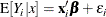In weighted least squares estimation you seek the parameters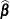that minimize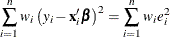where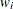is the weight associated with the ith observation. The normal equations of this minimization problem can be written as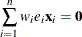In M-estimation the corresponding equations take on the form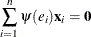where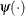is a weighing function. The Beaton-Tukey biweight, for example, can be written as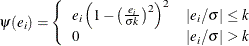Substitution into the estimating equation for M-estimation yields weighted least squares equations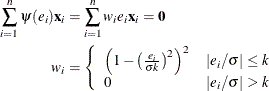The biweight function involves two constants,and k. The scalecan be fixed or estimated from the fit in the previous iteration. Ifis estimated, a robust estimator of scale is typically used. In this exampleis fixed at 2. A common value for the constant k is k = 4.685.

The following DATA step creates a SAS data set of the population of the United States (in millions), recorded at 10-year intervals starting in 1790 and ending in 1990. The aim is to fit a quadratic linear model to the population over time.

title 'U.S. Population Growth';
data uspop;
input pop :6.3 @@;
retain year 1780;
year   = year+10;
yearsq = year*year;
datalines;
3929 5308 7239 9638 12866 17069 23191 31443 39818 50155
62947 75994 91972 105710 122775 131669 151325 179323 203211
226542 248710
;


The PROC NLIN code that follows fits this linear model by M-estimation and IRLS. The weight function is set to a zero or nonzero value depending on the value of the scaled residual. The NOHALVE option removes the requirement that the (weighted) residual sum of squares must decrease between iterations.

title 'Beaton/Tukey Biweight Robust Regression using IRLS';
proc nlin data=uspop nohalve;
parms b0=20450.43 b1=-22.7806 b2=.0063456;
model pop=b0+b1*year+b2*year*year;
resid = pop-model.pop;
sigma = 2;
k     = 4.685;
if abs(resid/sigma)<=k then _weight_=(1-(resid / (sigma*k))**2)**2;
else _weight_=0;
output out=c r=rbi;
run;


Parameter estimates from this fit are shown in Output 69.2.1, and the computed weights at the final iteration are displayed in Output 69.2.2. The observations for 1940 and 1950 are highly discounted because of their large residuals.

Output 69.2.1: Nonlinear Least-Squares Analysis

 Beaton/Tukey Biweight Robust Regression using IRLS

The NLIN Procedure

Source DF Sum of Squares Mean Square F Value Approx
Pr > F
Model 2 113564 56782.0 49454.5 <.0001
Error 18 20.6670 1.1482
Corrected Total 20 113585

Parameter Estimate Approx
Std Error
Approximate 95% Confidence
Limits
b0 20828.7 259.4 20283.8 21373.6
b1 -23.2004 0.2746 -23.7773 -22.6235
b2 0.00646 0.000073 0.00631 0.00661

Output 69.2.2: Listing of Computed Weights from PROC NLIN

Obs pop year yearsq rbi sigma k _weight_
1 3.929 1790 3204100 -0.93711 2 4.685 0.98010
2 5.308 1800 3240000 0.46091 2 4.685 0.99517
3 7.239 1810 3276100 1.11853 2 4.685 0.97170
4 9.638 1820 3312400 0.95176 2 4.685 0.97947
5 12.866 1830 3348900 0.32159 2 4.685 0.99765
6 17.069 1840 3385600 -0.62597 2 4.685 0.99109
7 23.191 1850 3422500 -0.94692 2 4.685 0.97968
8 31.443 1860 3459600 -0.43027 2 4.685 0.99579
9 39.818 1870 3496900 -1.08302 2 4.685 0.97346
10 50.155 1880 3534400 -1.06615 2 4.685 0.97427
11 62.947 1890 3572100 0.11332 2 4.685 0.99971
12 75.994 1900 3610000 0.25539 2 4.685 0.99851
13 91.972 1910 3648100 2.03607 2 4.685 0.90779
14 105.710 1920 3686400 0.28436 2 4.685 0.99816
15 122.775 1930 3724900 0.56725 2 4.685 0.99268
16 131.669 1940 3763600 -8.61325 2 4.685 0.02403
17 151.325 1950 3802500 -8.32415 2 4.685 0.04443
18 179.323 1960 3841600 -0.98543 2 4.685 0.97800
19 203.211 1970 3880900 0.95088 2 4.685 0.97951
20 226.542 1980 3920400 1.03780 2 4.685 0.97562
21 248.710 1990 3960100 -1.33067 2 4.685 0.96007

You can obtain this analysis more conveniently with PROC ROBUSTREG. The procedure re-estimates the scale parameter robustly between iterations. To obtain an analysis with a fixed scale parameter as in this example, use the following PROC ROBUSTREG statements:

proc robustreg data=uspop method=m(scale=2);
model pop = year year*year;
output out=weights weight=w;
run;

proc print data=weights;
run;


Note that the computation of standard errors in the ROBUSTREG procedure is different from the calculations in the NLIN procedure.